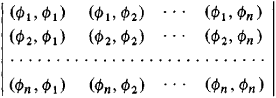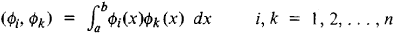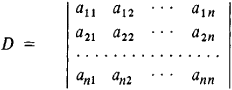# Linear Dependence

Also found in: Dictionary, Wikipedia.

## linear dependence

[′lin·ē·ər di′pen·dəns]
(mathematics)
The property of a set of vectorsv, …,vn in a vector space for which there exists a linear combination such that a1v1+ ··· + an vn = 0, and at least one of the scalars ai is not zero.

## Linear Dependence

(mathematics), a relationship of the form

(*) C1u1 + C2u2 + ... + Cnun = 0

where C1, C2,..., Cn are numbers, at least one of which is not zero, and u1, u2,..., un are various mathematical objects for which the operations of addition and multiplication by a number are defined. The dependence relation (*) is said to be linear in the objects u1, u2,..., un, since each of them enters (*) linearly, that is, the degree of each ui in (*) is one. The equality sign in the above relation may have various meanings and its sense must be specified in each particular case.

The concept of linear dependence is used in many branches of mathematics. We may thus speak, for example, of linear dependence between vectors, between functions of one or several variables, and between elements of a vector space. If the objects u1, u2,..., un are connected by a relation of linear dependence, then we say that they are linearly dependent. In the opposite case we say that they are linearly independent. If the objects u1, u2,..., un are linearly dependent, then at least one of them is a linear combination of the others, that is,

ui = α1u1 + ... + αi - 1ui-1 + αi + 1ui + 1 + ... + αnun

Continuous functions of one variable

u1 = ϕ1(x), u2 = ϕ2(x), . . ., un = ϕn (x)

are said to be linearly dependent if they are connected by a relation of the form (*), in which the equality sign is understood as an identity in x. In order for the functions Φ1(x), Φ2(x), . . ., Φn(x) defined on an interval axb to be linearly dependent, it is necessary and sufficient that their Gramian vanish, the Gramian in this case being the determinantWhereHowever, if the functions Φ1(x), Φ2(x),..., Φn(x) are solutions of a linear differential equation, then they are linearly dependent if and only if their Wronskian vanishes at at least one point.

Linear forms in M variables

ui = ai 1x1 + ai 2x2 + ... + aimxmi = 1, 2,..., n

are said to be linearly dependent if they are connected by a relation of the form (*), in which the equality sign is understood as an identity in all the variables x1, x2,..., xm. In order for n linear forms in n variables to be linearly dependent, it is necessary and sufficient that the following determinant vanish:References in periodicals archive ?
For a range of very low analyte density with a molecular coverage from 0.2% to 6% on the substrate surface; the SERS signal of the analytes exhibits a linear dependence on the molecule packing density on a logarithmic scale, with a slope of about 1.25.
In this case D becomes variable and one deals with the manifestation of a piecewise linear dependence of time.
Figure 9 shows that the experimental values of [lambda] of the FE-Fe and POM-Fe composites are in agreement with the linear dependence calculated from Eq 9 at N = 1 (Table 2).
The residuals from Equation (6) were then checked for any remaining linear dependence. Table 3 gives the autocorrelations for them alongwith the Box-Pierce Q statistic.
Even more significant is the fact that the linear dependence of the lattice spacing with gallium concentration implied that homogeneous Cu[In.sub.1-x][Ga.sub.x][Se.sub.2] alloys were produced for values of x between 0 and 0.35.
Linear dependence of the business process model scope and its application in management was studied by means of a correlation analysis between a set of business process items and a set of possibilities for its real application to meet the needs of managers.
Each analog input signal converted into power units using the linear dependence
After the 10th step of the punch displacement h the change of the angle [alpha] changes its linear dependence to nonlinear.
However, the use of the method of "cutting" established linear dependence t--[DELTA]1log VB and overcome this problem.
The new Hubble relation of QCM reduces to the linear dependence of the standard Hubble relation for z small, agreeing with there being no acceleration presently.
In a similar experiment  when nanocrystalline Fe was prepared using the technique of mechanical attrition, measurements of grain growth revealed a linear dependence of the grain size on annealing time, contradicting studies in coarser-grained materials, which had a parabolic (or power-law) dependence.

Site: Follow: Share:
Open / Close